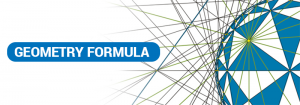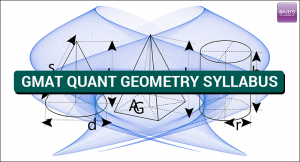# GMAT Quantitative#### GMAT Quant: Geometry Formula

For any Maths and Quantitative Aptitude sum, formulas are those fundamentals which help you to resonate and solve it. In …

#### Arithmetic Preparation Tips for GMAT

The elementary branch of mathematics is Arithmetic. It is a method or process of computation with figures includes addition, subtraction, …#### GMAT Quant Geometry Syllabus

GMAT test is intended to evaluate your critical thinking skills, the capability to interpret the problem and solving it. If …#### GMAT Quant Arithmetic Syllabus

GMAT Quant section comprises questions which involve arithmetic to certain extent. This test section follows an algorithm with every question …#### GMAT Quant: Vedic Maths Tips and Tricks

For International MBA, GMAT is the only key to unlock the gates for world’s renowned B-schools. And the quant section …